Department of Pre-University Education, KarnatakaPUC Karnataka Science Class 11

# Concept of Work

#### notes

Scientific concept of work:

• To understand the way we view work and define work from the point of view of science, let us consider some situations:
• Push a pebble lying on a surface. The pebble moves through a distance. You exerted a force on the pebble and the pebble got displaced. In this situation work is done.
• A girl pulls a trolley and the trolley moves through a distance. The girl has exerted a force on the trolley and it is displaced. Therefore, work is done.
• Lift a book through a height. To do this you must apply a force. The book rises up. There is a force applied on the book and the book has moved. Hence, work is done.

A closer look at the above situations reveals that two conditions need to be satisfied for work to be done:

(i) a force should act on an object, and

(ii) the object must be displaced.

If any one of the above conditions does not exist, work is not done. This is the way we view work in science.

### Work:

“The work done by the force is defined to be the product of component of the force in the direction of the displacement and the magnitude of this displacement.”

The work W done by a constant force F when its point of application undergoes a displacement s is defined to be
W=bar F.bar s=F s cos θ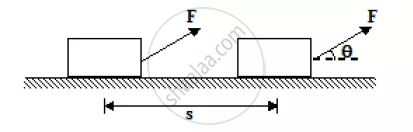where θ is the angle between bar F and bar s as indicated in figure. Only the component of bar F along s, that is fcos θ, contributes to the work done.
Work is a scalar quantity and its SI unit is the joule (J). From the above equation,  we see that
1 J = 1 Nm = 107 erg
In terms of rectangular components, the two vectors are
bar F= F_x hat i + F_y hat j + F_ z hat k
and bar s = Δx hat i +Δ y hat j+Δ z hat k
hence, the above equation may be written as
W=F_x Δx + F_y Δy + F_z Δz

The work done by a given force on a body depends only on the force, the displacement, and the angle between them. It does not depend on the velocity or the acceleration of the body, or on the presence of other forces.

When several forces act on a body one may calculate the work done by each force individually. The net work done on the body is the algebraic sum of individual contributions.
W_"net" = bar F_1. bar S_1 + bar F_2. bar S_2 +.........+ bar F_n. bar S_n or
W_"net" = W_1 + W_2 +............ + W_n

No work is done if:

1. The displacement is zero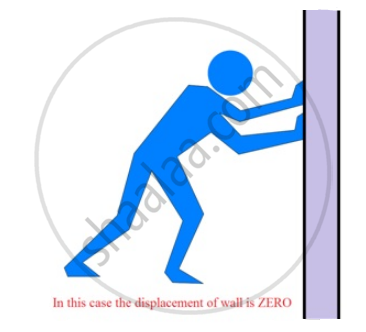2. The force is zero.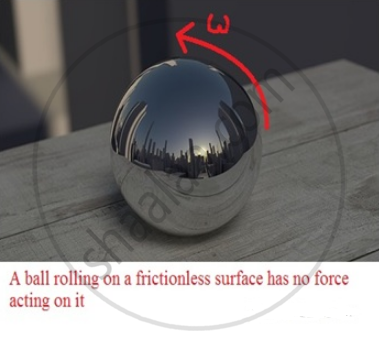3. The force and displacement are mutually perpendicular.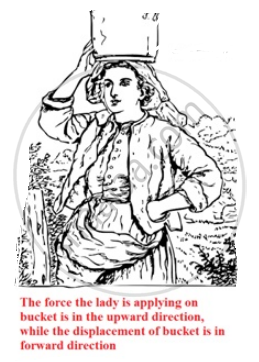Positive, Negative and Zero Work:-
Work done by a force may be positive or negative depending on the angle θ between the force and displacement. If the angle θ is acute (θ < 90^o), then the work done is positive and the component of force is parallel to the displacement.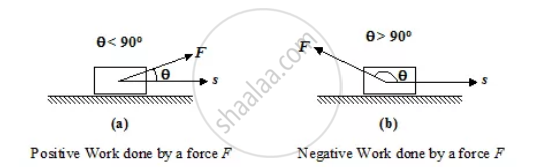If the angle θ is obtuse (θ > 90^o), the component of force is antiparallel to the displacement and the work done by force is negative.

Application 1
When a person lifts a body from the ground to some higher position, the work done by the lifting force (i.e. the force applied by the person) is positive since force (vector) and displacement (vector) are along the same (vertically upward) direction and hence,
θ = 0 cos θ =1
However, the work done by the gravity (or the force by the earth on the body) is negative since force (vector) and displacement (vector) are oppositely directed and hence
θ = 180o cos θ = −1.

Application 2
A box is moved over a horizontal path by applying force F = 60 N at an angle θ = 30o to the horizontal. What is the work done during the displacement of the box over a distance of 0.5 km.
Solution:
By definition, W = F s cos θ
Here F = 60 N; s = 0.5 km = 500m; θ= 30o
W = (60)(500) cos30o = 26 kJ

#### notes

Let a constant force, F act on an object. Let the object be displaced through a distance, s in the direction of the force. Let W be the work done. We define work to be equal to the product of the force and displacement. Work done = force × displacement

W = F s       ..(1)

Thus, work done by a force acting on an object is equal to the magnitude of the force multiplied by the distance moved in the direction of the force. Work has only magnitude and no direction. in Eq.(1), if F = 1 N and s = 1 m then the work done by the force will be 1 N m. Here the unit of work is newton metre (N m) or joule  (J).  Thus 1 J is the amount of work.

#### notes

Work (W)

Definition of Work Done: Work is defined as the product of the force applied on an object and displacement caused due to the applied force in the direction of the force. Work is a scalar quantity. It has no direction of its own but a magnitude.

It is expressed as the product of force and displacement in the direction of force.
W=F x s
Here W= work done on an object
F = Force on the object
s = Displacement of the object
The unit of Work is Newton metre (Nm) or joule (J).
1 Joule is defined as the amount of work done by force of 1 N when displacement is 1 m.

#### description

• Definition of work
• Units of work
• Relationship between joule and erg
• Positive, Negative, and Zero Work
If you would like to contribute notes or other learning material, please submit them using the button below.

### Shaalaa.com

Introduction to Work and Numericals [00:22:46]
S
0%